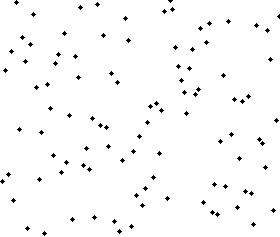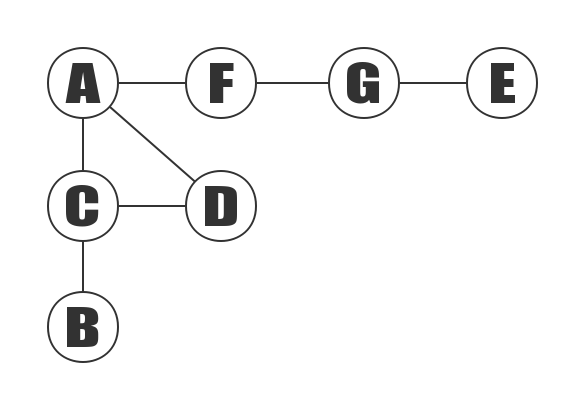# SSE Instruction List

## move instruction

Instruction Description
movaps move 4 alignment single precision value to xmm register
movups move 4 non-alignment single precision value to xmm register
movss move 1 alignment single precision value to low 4 bytes of register
movlps move 2 alignment single precision value to low 8 bytes of register
movhps move 2 alignment single precision value to high 8 bytes of register
movlhps move 2 alignment single precision value to high 8 bytes of register from low 8 bytes
movhlps move 2 alignment single precision value to low 8 bytes of register from high 8 bytes

## basic operation instruction

Instruction Description
subps sub operation
mulps mul operation
divps div operation
rcpps rcp opeartion
sqrtps sqrt operation
rsqrtps rcp sqrt operation
maxps get max operation
minps get min operation
andps and operation
andnps negation operation
orps or operation
xorps xor operation

## compared instruction

Instruction Description
cmpps compared operation
cmpss compared operation
comiss compared and set eflags register
ucomiss compared and set eflags register

those instruction will return a value:

Return Value Description
0 Equal to
1 Less-than
2 Less than or equal to
3 Disorder
4 Not equal to
5 Greater than
6 Greater than or equal to
7 Order
Share

# 8 Kind of Sorting Algorithm

## Bubble sortShare

# N-Queens Problem

## Overview

The eight queens problem is a question with chess as the background: how to place eight queens on an 8×8 chess board so that no queen can directly eat other queens? In order to achieve this purpose, any two queens are not in the same horizontal, vertical or diagonal. The eight queen problem can be extended to the more general N-Queens Problem.

Share

# Topological Sort

## Overview

Topological sorting means ordering a Directed Acyclic Graph(DAG) to get an ordered linear sequence.

In this way, it may be understood more abstractly.

For example, a project consists of four subsections A, B, C, and D, and A depends on B and D. C depends on D. Now to develop a plan to write A, B, C, D the order of execution. At this point, you can use topological sorting, which is used to determine the order in which things happen.

In topological sorting, if there is a path from the vertex A to the vertex B, B appears behind the A in the ranking result.

## Algorithm Introduction

1. Create a queue Q and a topological ordered result queue T.
2. Put all the nodes that do not depend on the vertex in the Q.
3. When Q has vertices, perform the following steps.
1. Take a vertex n from Q (delete n from Q) and put it in T (add n to the result set).
2. For every adjacent point m (n is the starting point and m is the ending point).
1. Remove the edge (n, m).
2. If m does not depend on the vertex, put b into Q.

The vertex A does not depend on the vertex, which means there is no edge to the end of the A.

Share

## Overview

The breadth first search algorithm, also known as “width first search” or “horizontal priority search”, is called BFS.

From a vertex in the graph, each of the not visited adjacent points of the V is accessed in turn after accessing the starting vertex, then the adjacency points are accessed in turn from these adjacent points, and the adjacent points of the vertex to be accessed are accessed before the adjacent points of the vertices that are accessed, until all the adjacent points of the vertices are accessed. If the vertices are not accessed at this time, we need to select another vertex which has never been visited as a new starting point, repeat the above process until all the vertices in the graph are accessed.

In other words, the process of breadth first search is the starting point and from near to far, access to the path and the path length of the starting vertex and the path length of 1,2…

## Diagram

### Breadth first search for undirected graphs

The following is an example of undirected graph, to demonstrate the depth-first search.Share

# Depth First Search

Depth First Search Chinese version address

# Depth First Search

## Overview

Depth first search of the graph is similar to the preorder traversal of the tree.

Suppose that the initial state is that all the vertices in the graph are not accessed, then starting from a vertex and first access to it, nextly, the depth first search traversal from each of its non-accessed adjacency points in turn, until all the vertices that have a path to the starting vertex in the graph are accessed. If there are other vertices that are not accessed, then choose another unvisited vertex as a starting point, repeat the above process until all graph vertices have been visited.

## Diagram

### Depth first search for undirected graphs

The following is an example of undirected graph, to demonstrate the depth first search.Share

Adjacency matrix undirected graph refers to an undirected graph represented by an adjacency list.The above graph contains 7 vertices of A, B, C, D, E, F, G, and it also contains<A,C>, <A,D>, <A,F>, <B,C>, <C,D>, <E,G>, <F,G>, in total 7 edges. Since this is an undirected graph, the edge <A,C> and the edge <C,A> are the same edges. The table of edges is listed in alphabetical order..

Share

Adjacency matrix undirected graph refers to an undirected graph represented by an adjacency matrix.The above graph contains 7 vertices of A, B, C, D, E, F, G, and it also contains, , , , , , , in total 7 edges. Since this is an undirected graph, the edge and the edge are the same edges. The table of edges is listed in alphabetical order.

Share

# Basic Graph Theory

Basic Graph Theory Chinese version address

# Graph Theory

## The definition of graph

The graph is composed of some points and the connection between these points. points are often called vertices, and the lines between points are called edges. It is usually written as G = (V,E).

### Types of Graphs

According to whether the direction of the edge, the graph can be divided into: undirected and directed graph.

#### Undirected graphShare